Electron. J. Diff. Eqns., Vol. 2000(2000), No. 65, pp. 1-8.

### Positive solutions to a second order multi-point boundary-value problem Daomin Cao & Ruyun Ma

Abstract:
We prove the existence of positive solutions to the boundary-value problem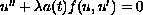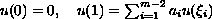,
whereis a continuous function that may change sign on [0,1],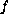is a continuous function with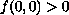, and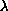is a samll positive constant. For finding solutions we use the Leray-Schauder fixed point theorem.

Submitted September 18, 2000. Published October 30, 2000.
Math Subject Classifications: 34B10.
Key Words: Multi-point boundary value problem, positive solution, fixed point theorem.

Show me the PDF file (108K), TEX file, and other files for this article.Daomin Cao Institute of Applied Mathematics Academy of Mathematics and System Sciences Beijing 100080, People's Republic of China email: cao@amath6.amt.ac.cnRuyun Ma Department of Mathematics Northwest Normal University Lanzhou 730070, Gansu, P. R. China email: mary@nwnu.edu.cn